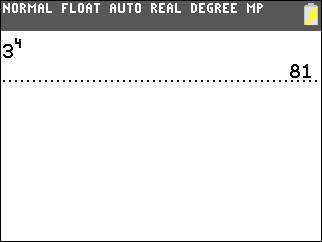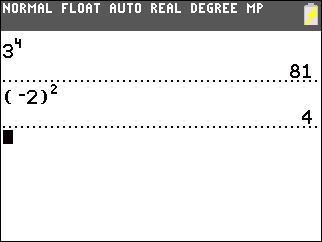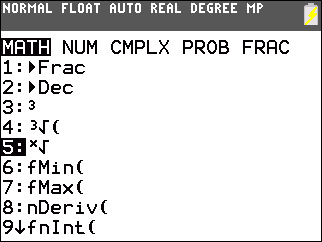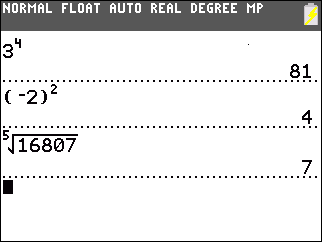# Knowledge Base

## Solution 34754: Calculating nth Powers and nth Roots on the TI-83 Plus and TI-84 Plus Family of Graphing Calculators.

### How do I calculate nth powers and nth roots on the TI-83 Plus and TI-84 Plus family of graphing calculators?

To calculate nth powers and nth roots using the on the on the TI-83 Plus and TI-84 Plus family of graphing calculators, follow the examples below:

nth Powers

To raise a number by an exponent, use the caret key labeled as [^].

Example: 34 = ?

• Press .
• Press [^].
• Press .
• Press [ENTER] to complete the calculation.Example: -22 = ?

• Press [ ( ] [(-)]. The [(-)] key is called the negate key and is located below .
• Press  [ ) ].
• Press [^] .
• Press [ENTER] to complete the calculation.

NOTE: Since the handheld evaluates the negate symbol as multiplying the value by (-1), and exponents come before multiplication using the Order of Operations, the handheld will evaluate -22 as (-4). Thus, negative values will need to be enclosed in parentheses to compute the desired solution of 4.nth Roots

To find the nth root of a number, use the nth root command (x√). To input the nth root command, press [MATH] .

Example: Find the 5th root of 16807.

• Press .
• Press [MATH] .• Press     .
• Press [ENTER] to complete the calculation.Please see the TI-83 Plus and TI-84 Plus Family guidebooks for additional information.